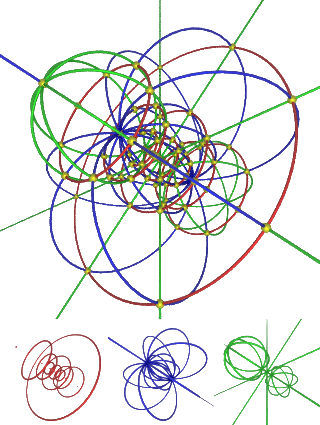# Hypersphere Packing

Geometry Level 4Consider a line segment of length 2. Obviously, 2 unit segments (of length 1) can fit in it. Likewise, 4 unit squares can fit into a 2 by 2 square, and 8 unit cubes can fit into a 2 by 2 by 2 cube.

Doing the same with spheres, we see that 4 unit spheres fit in a 2 by 2 square, 8 unit spheres inside a 2 by 2 by 2 cube, etc.

How many unit hyperspheres can you fit in a 2 by 2 by 2 by 2 hypercube?

Note: In the context of this question, it is inferred that a "unit hypersphere" has a diameter of 1. The standard definition is that a "unit hypersphere" has a radius of 1.

×